# High School Math : How to find the perimeter of a rhombus

## Example Questions

### Example Question #677 : High School Math

This figure is a rhombus with a side of 8 in.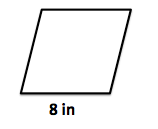What is the perimeter of the rhombus (in)?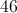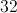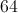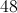Explanation:

The perimeter of the rhombus is the distance around the outside of the figure.  Since a rhombus has 4 congruent sides, the perimeter is simply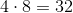.

### Example Question #678 : High School Math

You are given a rhombus with a side ofmeters. What is the perimeter of the rhombus?metersmetersmetersmetersmetersmeters
A rhombus is a quadrilateral (sided figure) withcongruent sides. The perimeter is the sum of the sides. Thus, to find the perimeter of the rhombus, we multiply the perimeter by. This gives usmeters.